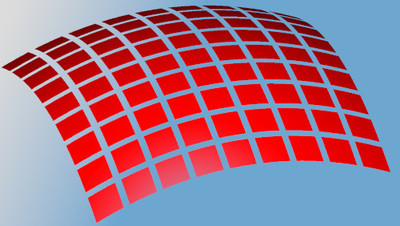# Multidimensional Calculus

used at University of Rochester

Educator Edition

 Course Multidimensional Calculus: Equations of lines and planes, quadric surfaces, space curves, partial derivatives, linear approximation, directional derivatives, extrema, Lagrange multipliers, double/triple integrals including cylindrical and spherical coordinates. Line, surface, and volume integrals, divergence theorem, Stokes' theorem. Textbook This course is frequently paired with the following textbook(s): Calculus: Early Transcendentals (8th edition or earlier) by James Stewart

Edfinity is a full-featured homework system. Use this problem series as-is, or mix-and-match with other series or your own problems as desired. Edfinity gives you complete control over every aspect of students' online assessment experience.

## Powered by WeBWorK

This problem series includes WeBWorK-enhanced problems. WeBWorK problems are highly sophisticated, supporting the representation and manipulation of mathematical objects such as vectors, points, matrices, intervals, and complex numbers, with customizable answer checkers. Students are encouraged to make multiple attempts until they succeed, and given immediate feedback on correctness.

Edfinity provides full access to the WeBWorK Open Public Library (OPL), a collection of over 20,000 rich, interactive problems across a wide range of math and science subjects, including college algebra, discrete mathematics, probability and statistics, single and multivariable calculus, differential equations, linear algebra and complex analysis.

## Problem Sets

1. Edfinity Demo
2. Three-Dimensional Coordinate Systems; Vectors; Dot Product
3. Dot Product; Cross Product; Equations of Lines and Planes
4. Quadric Surfaces and Cylinders; Vector Functions and Space Curves; Derivatives and Integrals of Space Curves
5. Arc Length and Curvature; Motion in Space; Functions of Several Variables
6. Functions of Several Variables; Limits and Continuity; Partial Derivatives
7. Partial Derivatives
8. Maximum and Minimum Values; Lagrange Multipliers
9. Multiple Integrals; Iterated Integrals
10. Double Integrals over General Regions; Change of Variables in Multiple Integrals; Polar Coordinates
11. Integration in Polar Coordinates; Applications of Double Integrals; Triple Integrals; Integrals in Spherical Coordinates
12. Vector Fields; Line Integrals; FTC for Line Integrals Against Gradient Fields; Green's Theorem
13. Green's Theorem; Curl and Div; Parametric Surfaces
14. Surface Integrals; Stoke's Theorem; Divergence Theorem

##License

This is a multi-student, educator license. You will be able to manage an online course with your students, and monitor their progress.

Price: FREE
Trusted by## Try problems from this course

Cookies help us deliver our services. By using our services, you agree to our use of cookies.